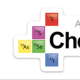# Updated 2015 AP Chemistry InfographicFebruary 03, 2015

1.Can a student miss 10 MC questions or are 10 MC questions not counting towards the score?

•Hi Suzie

Here is what it means;

Students should attempt to answer ALL of the 60 of the questions, but only 50 of them actually count toward the final score – the other 10 questions are used for statistical analysis only, and are NOT counted in the scoring of the MCQ section. Obviously, students don’t know which ones are counted and which are ignored, so it makes absolutely ZERO practical difference to them and they should attempt all questions as if they DO count.

In 2014, the MCQ approx. score boundaries were (out of 50), these; 5 = 38, 4 = 31, 3 = 24, 2 = 17.

BUT far more important are the overall %’s. In 2014, overall %’s and grade boundaries were these; 5 = 72%, 4 = 58%, 3 = 42%, 2 = 27%.